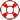Standard:

# 8.9.A

Description:

## identify and verify the values of x and y that simultaneously satisfy two linear equations in the form y = mx + b from the intersections of the graphed equations.8th Grade Math - Systems of Equations Lesson# Processing Request...